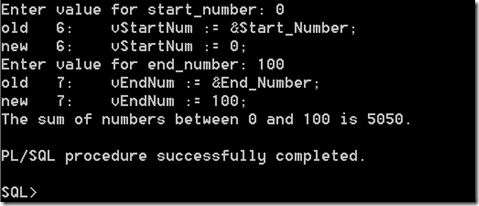## Tuesday, November 6, 2012

### Adding numbers between two values

My son asked me the other day, what is the total number if you add all the numbers between Zero and 100.
We could have sat down to work it out with some paper and a pen, but instead I decided to introduce him to the world of Oracle, SQL and PL/SQL
The first step we took was to work out how you would do it on paper for some of the numbers. Then we translated this into some PL/SQL code. OK I did a lot this but he did seem to understand and follow what I was doing.
So the following Function is what we ended up with to add all the numbers between two numbers and return the answer.
( pStartNum IN NUMBER,
pEndNum IN NUMBER)
RETURN NUMBER
IS
vSum   Number := 0;
BEGIN
FOR i IN pStartNum .. pEndNum LOOP
vSum := vSum + i;
END LOOP;
return vSum;
END;
/

The next step was to write some code to call this function. The code prompts the user to enter the Start number and End number.
set serveroutput on
DECLARE
vStartNum  NUMBER := 0;
vEndNum   NUMBER := 100;
BEGIN
vStartNum := &Start_Number;
vEndNum := &End_Number;